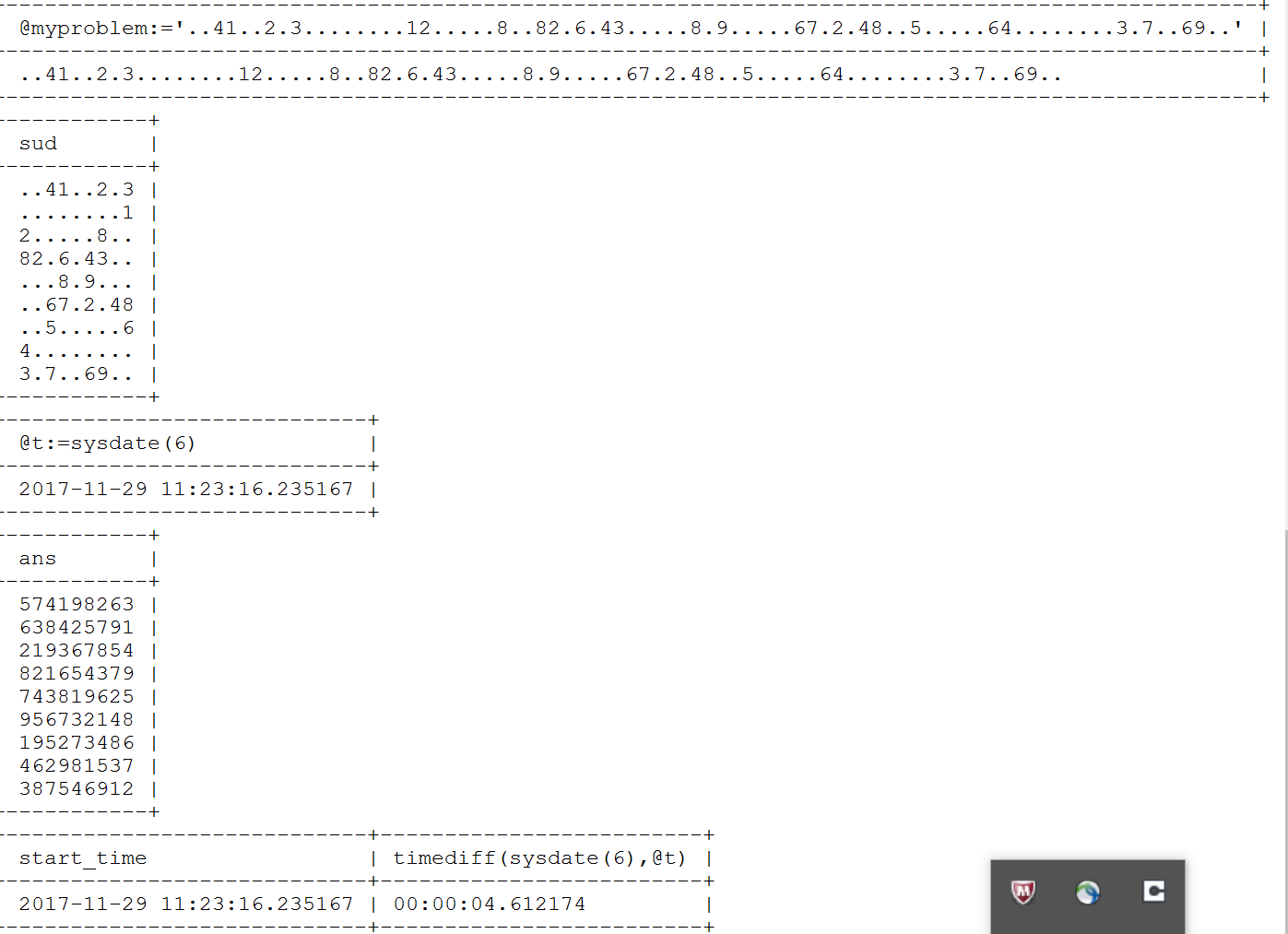## Tuesday, November 28, 2017

### SUDOKU Solver ｂａｓｅｄ　ｏｎ　ＣＴＥ　ｆｒｏｍ　ＭｙＳＱＬ　８.０

SUDOKU is an interesting problem.   Using SQL to solve this problem is not anything magical.

The following SQL with MySQL 8.0.3 RC1, the New Feature "Recursive Common Table Expression/CTE" enables the easy way of this SUDOKU solver.

select @myproblem:='..41..2.3........12.....8..82.6.43.....8.9.....67.2.48..5.....64........3.7..69..';

WITH RECURSIVE
my19(n) AS (
SELECT 1 AS n
UNION ALL
SELECT 1+n FROM my19 WHERE n<9
)
select substr(@myproblem,(n-1)*9+1,9) as sud from my19;

select @t:=sysdate(6);

WITH RECURSIVE
input(sud) as (
select @myproblem
),
digits(z,lp) as (
select '1', 1
union all
select cast(lp+1 as char), lp+1 from digits where lp<9
),
x(s,ind) as (
select sud, instr(sud,'.') from input
union all
select concat(substr(s,1, ind-1), z, substr(s, ind+1)),
instr( concat(substr(s,1,ind-1), z, substr(s, ind+1)), '.')
from x, digits as z
where ind> 0
and not exists (
select 1 from digits as lp
where z.z = substr(s, ((ind-1) div 9) *9 + lp, 1)
or z.z = substr(s, ((ind-1)%9) + (lp-1) *9 + 1, 1)
or z.z = substr(s, (((ind-1) div 3) %3) * 3
+ ((ind-1) div 27 ) * 27 + lp
+ ((lp-1) div 3) * 6, 1)
)
),
my19(n) AS (
SELECT 1 AS n
UNION ALL
SELECT 1+n FROM my19 WHERE n<9
)

select substr(s,(n-1)*9 + 1,9) as ans from x,my19 where ind=0 ;

select @t as start_time, timediff(sysdate(6),@t);

The Output from the above SQL is shown as follows :